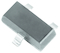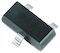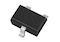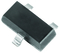Die and Wafer
Diodes and Rectifiers
Discrete Thyristors
IC - Power and Linear
MOSFETs
Optoelectronics
Power Modules
Capacitors
Resistors
Magnetics
Sensors
Other Components
Semiconductors
Passive Components
 My Vishay | Language

# Small Signal Diodes - Small signal switching diodes - SOT-23 Package

Small Signal Diodes - Small signal switching diodes - SOT-23 Package
Series
Product ImagePackage (JEDEC®) Code
VRRM
(V)
IR at VR
(nA at V)
VF at IF
(V at mA)
trr
(ns)
Circuit Configuration
AEC-Q101 QualifiedEnlarge
SOT-23 70 2500 at 70 0.715 at 1 6 Single YesEnlarge
SOT-23 70 2500 at 70 0.715 at 1 6 Single YesEnlarge
SOT-323 50 at 50 1.1 at 50 n/a Common cathode NoEnlarge
SOT-23 75 1000 at 75 0.715 at 1 6 Single YesEnlarge
SOT-23 75 1000 at 75 0.715 at 1 6 Single YesEnlarge
SOT-23 120 100 at 100 1.0 at 100 50 Single YesEnlarge
SOT-23 120 100 at 100 1.0 at 100 50 Single YesEnlarge
SOT-23 200 100 at 150 1.0 at 100 50 Single YesEnlarge
SOT-23 200 100 at 150 1.0 at 100 50 Single YesEnlarge
SOT-23 250 100 at 200 1.0 at 100 50 Single YesEnlarge
SOT-23 250 100 at 200 1.0 at 100 50 Single YesEnlarge
SOT-23 250 100 at 200 1 at 100 50 Common cathode YesEnlarge
SOT-23 250 100 at 200 1 at 100 50 Common cathode YesEnlarge
SOT-23 70 2500 at 70 0.715 at 1 6 Common cathode YesEnlarge
SOT-23 70 2500 at 70 0.715 at 1 6 Common cathode YesEnlarge
SOT-23 70 2500 at 70 0.715 at 1 6 Dual serial YesEnlarge
SOT-23 70 2500 at 70 0.715 at 1 6 Common anode YesEnlarge
SOT-23 70 2500 at 70 0.715 at 1 6 Common anode YesEnlarge
SOT-23 300 100 at 240 0.87 at 20 50 Common anode YesEnlarge
SOT-23 300 100 at 240 0.87 at 20 50 Common anode YesEnlarge
SOT-23 300 100 at 240 0.87 at 20 50 Common cathode YesEnlarge
SOT-23 300 100 at 240 0.87 at 20 50 Common cathode YesEnlarge
SOT-23 300 100 at 240 0.87 at 20 50 Dual serial YesEnlarge
SOT-23 300 100 at 240 0.87 at 20 50 Dual serial YesEnlarge
SOT-23 75 2500 at 70 1.0 at 10 4 Single YesEnlarge
SOT-23 75 2500 at 70 1.0 at 10 4 Single YesEnlarge
SOT-23 75 2500 at 70 0.72 at 5 4 Single YesEnlarge
SOT-23 75 2500 at 70 0.72 at 5 4 Single YesEnlarge
SOT-23 100 at 50 0.7 at 1 4 Single YesEnlarge
SOT-23 70 100 at 50 0.7 at 1 4 Single YesEnlarge
SOT-23 100 1000 at 50 0.70 at 1 4 Dual serial YesEnlarge
SOT-23 100 1000 at 50 0.70 at 1 4 Dual serial YesEnlarge
SOT-23 100 25 at 20 1 at 10 4 Single YesEnlarge
SOT-23 100 25 at 20 1 at 10 4 Single Yes